Examples

Chapter 15 Class 8 Introduction to Graphs
Serial order wise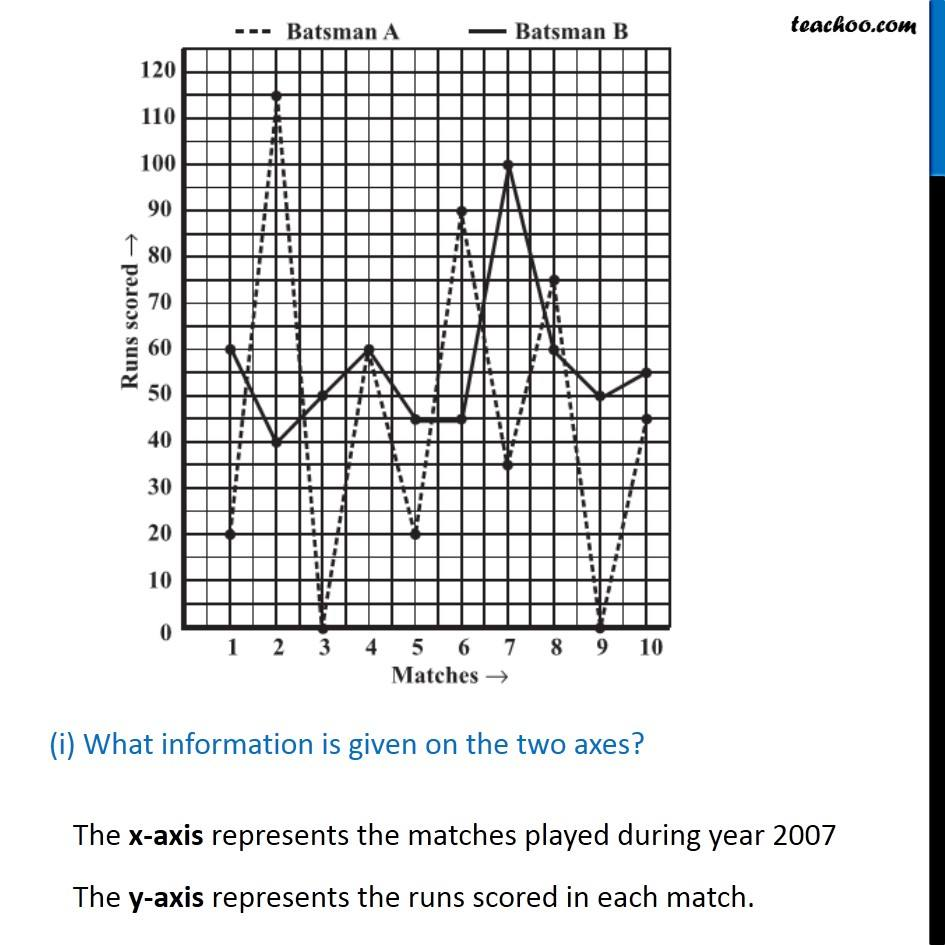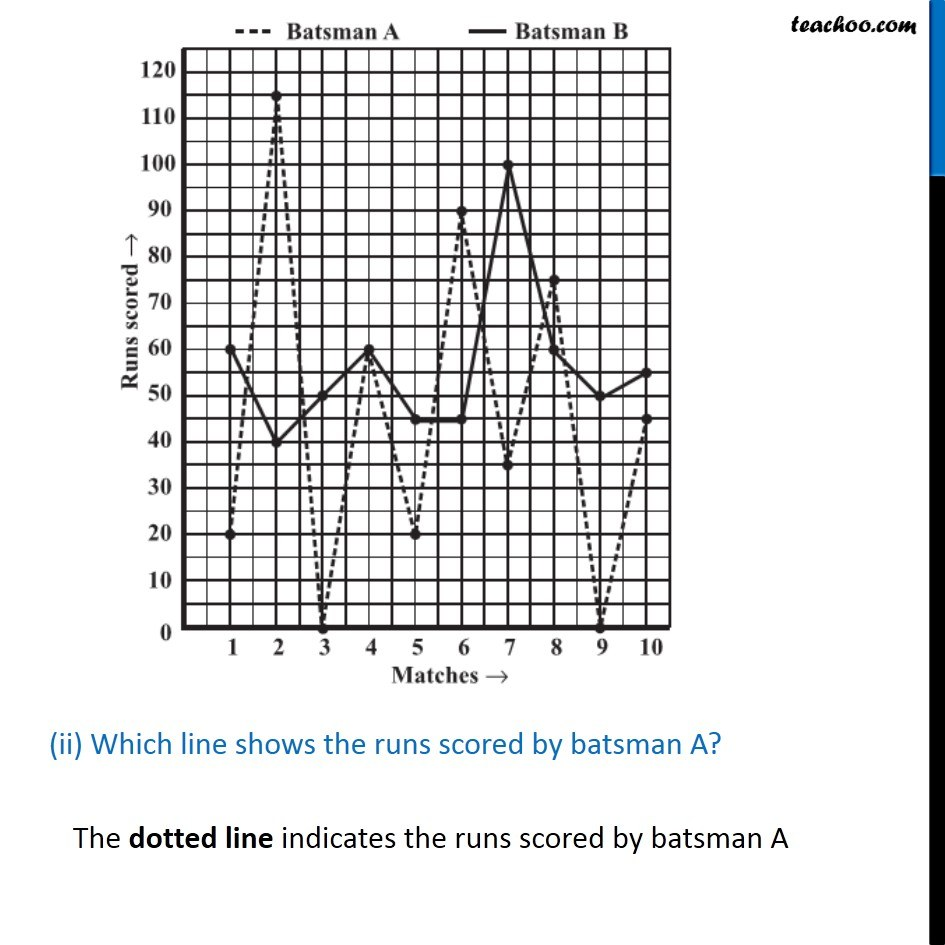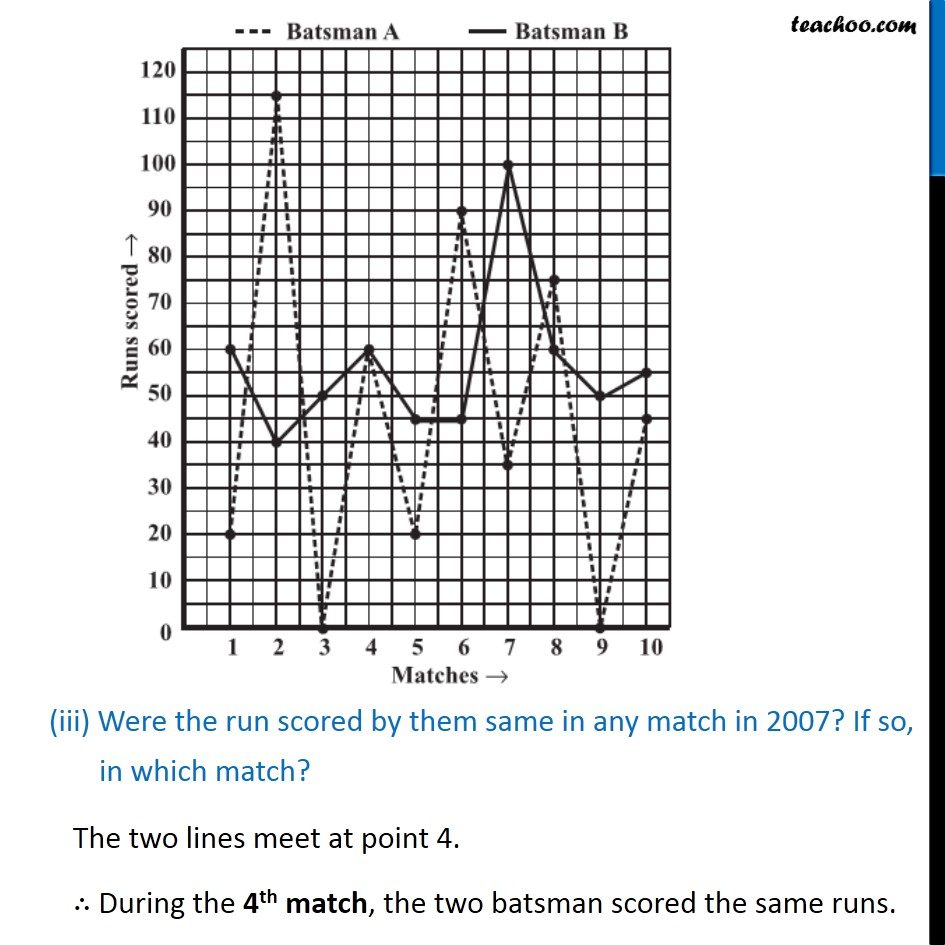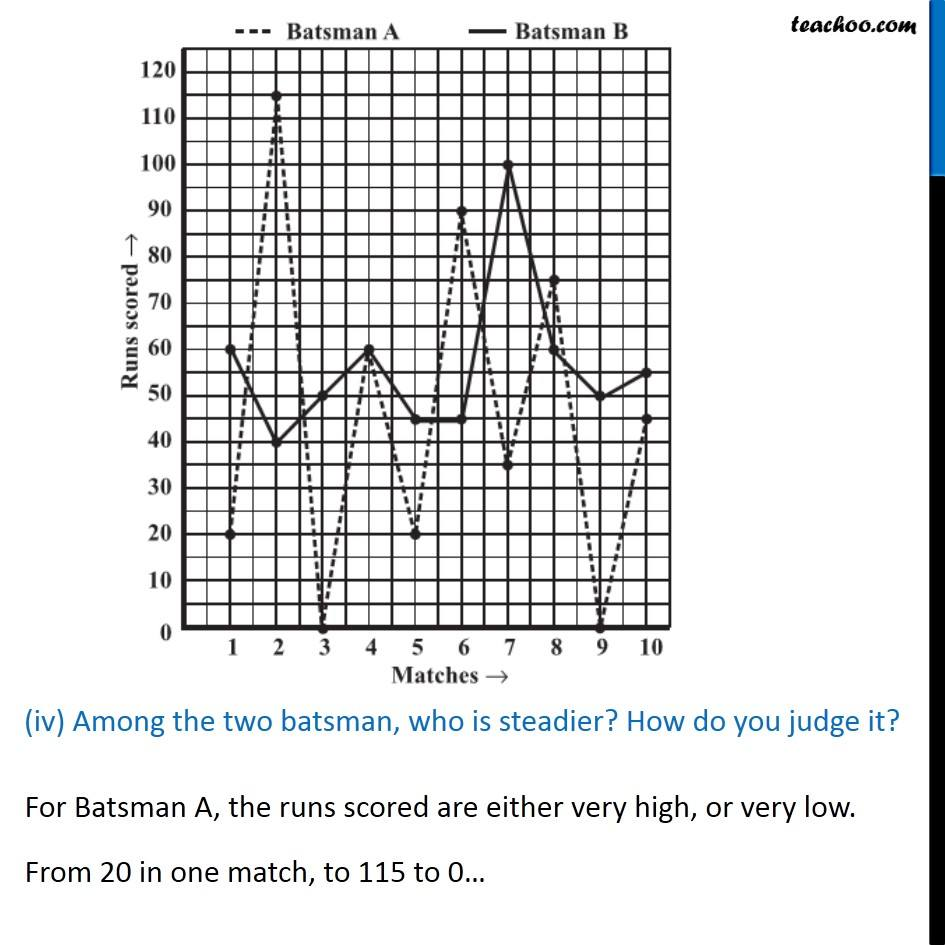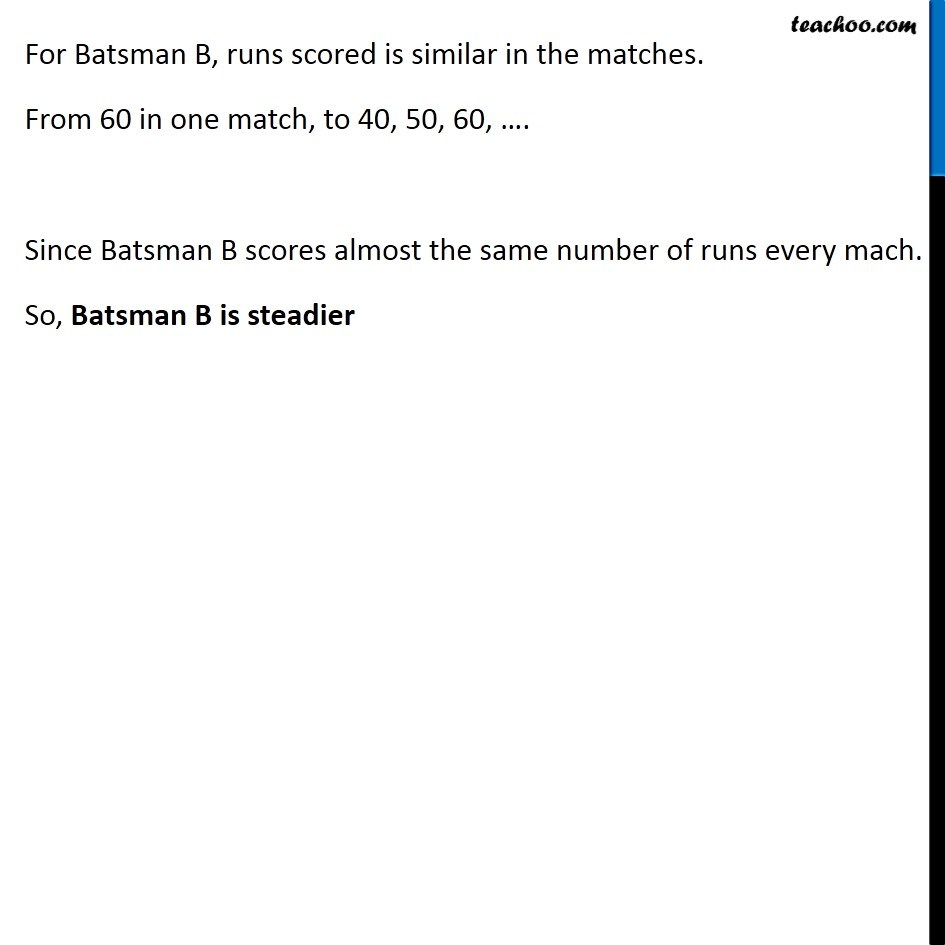### Transcript

Example 1 The given graph (Fig 15.7) represents the total runs scored by two batsman A and B, during each of the ten different matches in the year 2007. Study the graph and answer the following questions. (i) What information is given on the two axes? The x-axis represents the matches played during year 2007 The y-axis represents the runs scored in each match. (ii) Which line shows the runs scored by batsman A? The dotted line indicates the runs scored by batsman A (iii) Were the run scored by them same in any match in 2007? If so, in which match?The two lines meet at point 4. ∴ During the 4th match, the two batsman scored the same runs. (iv) Among the two batsman, who is steadier? How do you judge it? For Batsman A, the runs scored are either very high, or very low. From 20 in one match, to 115 to 0… For Batsman B, runs scored is similar in the matches. From 60 in one match, to 40, 50, 60, …. Since Batsman B scores almost the same number of runs every mach. So, Batsman B is steadier